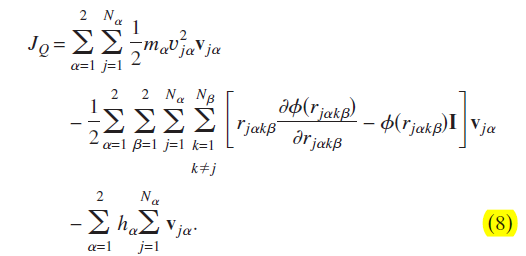# The calculation of heat current vector Jq of 2 component by lammps

Hi all users,
I want to simulate the Thermol Conductivity of 2 component of Cu-Ar by lammps, so I need to integrate the current autocorrelation function (HCACF) and at first, for example, I need to figer out how to get the data of the heat current vector Jq in x direction.
According to the fomula listed below, I need to figer out the last item ( if the system is single component, this item equals to zero) and subtract it from the Jq item, so I can get a right Jq in x direction, that is where the problem sets in, I was wondering how can i realise that by lammps, although described in ‘Molecular dynamics simulation of effective thermal conductivity in nanofluids’ in theorecitally, the last item of the formula(the average enthalpy ) can be calculated as the sum of the average kinetic energy, potential energy, and average virial terms per
particle of each species.I have not found the answer or some commands in lammps can calculate it, what i mean is that how to figer out the ‘h’ in the last itme of the formula.
I really appreciate for your kindly help.
Best regards.
PS: vj denotes the velocity of a particle j of kind , h denotes the average enthalpy per particle of species , and I is the unit tensor.I am not sure about your enthalpy term, but the heat flux as given by compute heat/flux () is usually suitable for determining conductivities by EMD.